# Color intersection between calculated column and calculated row

How to color intersection between calculations?

When you do calculations in TARGIT (calculated rows and columns), you end up with intersections where these calculations “meet”.

Here’s a crosstab showing revenue per Salesperson by Quarters with a calculated column and a calculated row, where the intersection is left blank.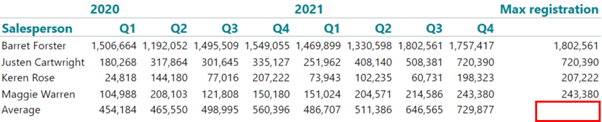The calculated column just picks out the maximum value from all the columns with this syntax:
max(all, 0, m1)

The calculated row calculates the average per salesperson with this syntax:
avg(0, all, m1)

To fill the intersection, you have to decide which calculation should continue – in this case the result will be different depending on which calculation is continued.

In this case we continue the calculated column, by opening up the Max registration calculation and picking calculate in intersection.

Now we tick the Average – which means that the max calculation will continue in the average row: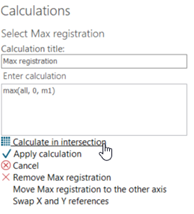Here’s the result – the max of the averages is picked out in the intersection cell: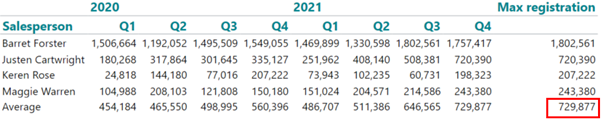So far so good.
Now we wan’t to color the Max registration column.

This can be done with a coloragent – just coloring all based on a condition that says all.

Here’s the result – and notice: the intersection cell is not included in the coloring.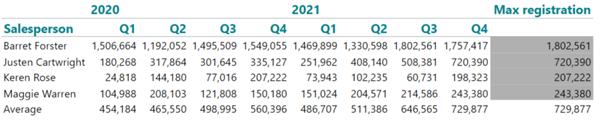Actually it’s not possible to color the intersection cell with a color agent.
It just can’t be reached this way.

So here’s creative approach – let’s to and change the crosstab theme for this particular crosstab.

PropertiesCrosstab Styling – coloring cells and alternating cells gives this result: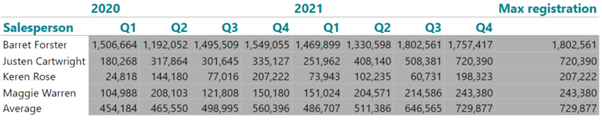Now we have the intersection cell included in the coloring – so now we just have to make 2 coloragents (one that colors revenue, and one that colors average) that gives a white background to all the other cells.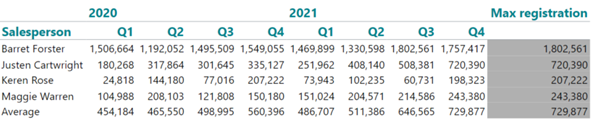And there we are – now the intersection cell is included in the coloring of the Max registration calculated column.

0 out of 0 found this helpful

• Hi Niels

Is it in meantime possible to add an icon were both row and column is calculated?

I use thumbs-up/down for my reports...

/ Lone

• Hi Lone

I understand your issue - but the intersection between a calculated column and a calculated row just can't be reached with a color agent.

However, there is a workaround, which I will show below.
The workaround has quite a few steps – and if you find this too cumbersome – I just want to make you aware that we've just opened up a new section for ideas in the community:

https://community.targit.com/hc/en-us/community/topics/7278561562141-Feature-requests-Ideas

It would be great if you could take the time to add this as an idea.
We review these ideas on a frequent basis.

Anyway – here’s the workaround.

I’m using the same table as before, and the same calculations – only difference is that the max registration in the workaround is calculated as a new measure: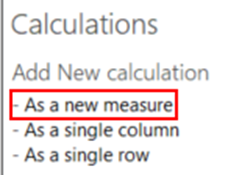This gives us the same result for each quarter (and most of these results are obviously redundant):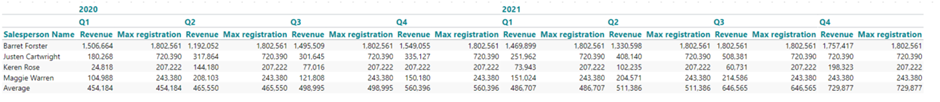However, by calculating as a new measure, we have better control of the intersection, and we can just hide the redundant Max registration columns by going to properties, visibility and hide  Max registration for a range of Year and Quarter: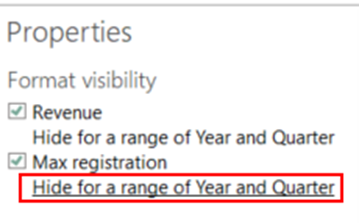Choose Position 1 from the first and then Define range end: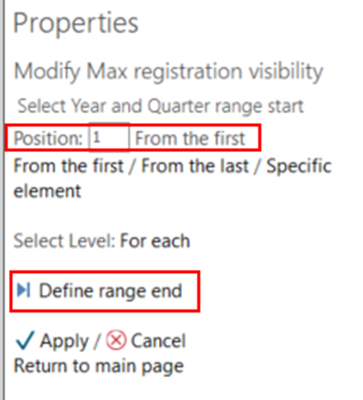Now choose Position 2From the lastApply: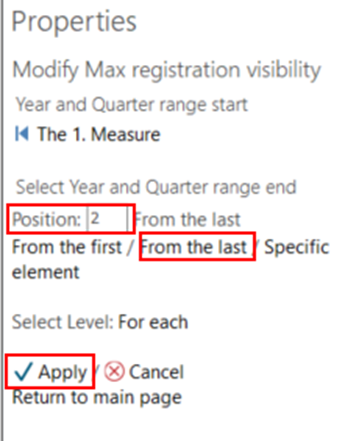Now the table looks as if we just calculated a column and not as a new measure: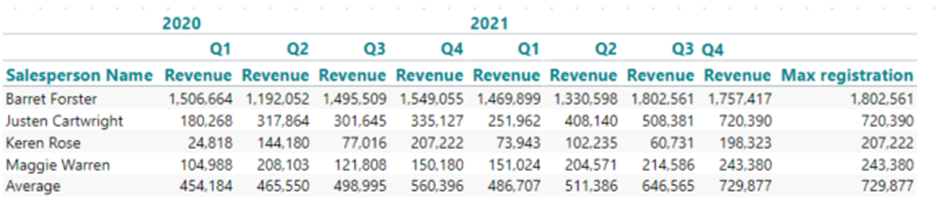Now when I setup my color agent to give max registrations above 700.000 an arrow up, I get this result: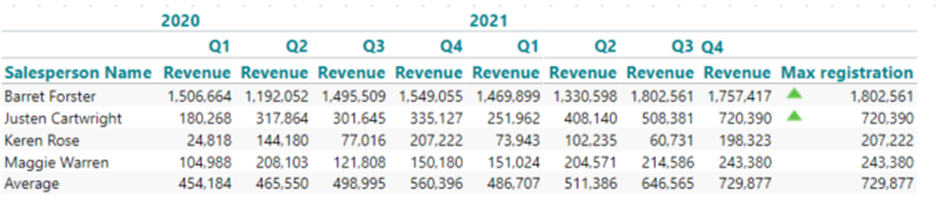It still doesn’t seem to reach the calculated row – we need to setup another color agent for the calculated row (Average) with this complex condition:

value > 700000 and allcount(d-1:0, 0, m1) = 1 and allcount(d-1, 0, m1:0) = 2

The syntax says the value has to be higher than 700.000, the first allcount makes sure that it only applies to the last column, and the second allcount makes sure that it only applies to the second measure (Max registration)

Result: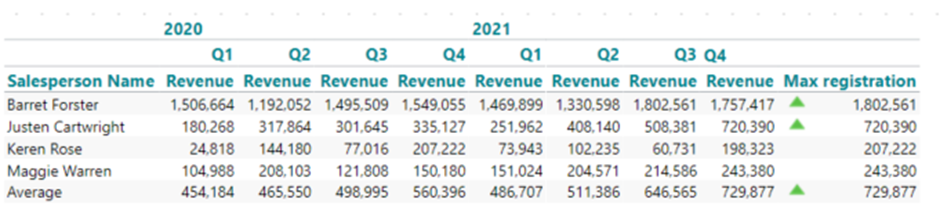Hope it makes sense.

Br/Niels